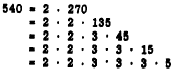Zero as a factorCustom SearchZERO AS A FACTOR If any number is multiplied by zero, the product is zero. For example, 5 times zero equals zero and may be written 5(0) = 0. The zero factor law tells us that, if the product of two or more factors is zero, at least one of the factors must be zero. PRIME FACTORS A number that has factors other than itself and 1 is a COMPOSITE NUMBER. For example, the number 15 is composite. It has the factors 5 and 3. A number that has no factors except itself and 1 is a PRIME NUMBER. Since it is sometimes advantageous to separate a composite number into prime factors, it is helpful to be able to recognize a few prime numbers quickly. The following series shows all the prime numbers up to 60: 2, 3, 5, 7, 11, 13, 17, 19, 23, 29, 31, 37, 41, 43,47,53, 59. Notice that 2 is the only even prime number. All other even numbers are divisible by 2. Notice also that 51, for example, does not appear in the series, since it is a composite number equal to 3 x 17. H a factor of a number Is prime, It is called a PRIME FACTOR, To separate a number into prime factors, begin by taking out the smallest factor. If the number is even, take out all the 2�s first, then try 3 as a factor, etc. Thus, we have the following example:Since 1 Is M understood factor of every number, we do not waste space recording It as one of the factors in a presentation of this kind, A convenient way of keeping track of the prime factor Is in the short division process as follows:If a number is odd, its factors will be odd numbers. To separate an odd number into prime factors, take out the 3�s first, if there are any. Then try 5 as a factor, etc. As an example, 5,775  =  3 x 1,925             =  3 x 5 x 385             =  3 x 5 x 5 x 77             =  3 x 5 x 5 x 7 x 11 Practice problems: 1. Which of the following are prime numbers and which are composite numbers ?     25, 7, 18, 29, 51 2. What prime numbers are factors of 36? 3. Which of the following are multiples of 3 ?      45, 53, 51, 39, 47 4.  Find the prime factors of 27. Answers: 1. Prime: 7, 29     Composite: 25, 18, 51 2. 36 = 2 x 2 x 3 x 3 3. 45, 51, 39 4. 27 = 3 x 3 x 3 Tests for Divisibility It is often useful to be able to tell by inspection whether a number is exactly divisible by one or more of the digits from 2 through 9. An expression which is frequently used, although it is sometimes misleading, is "evenly divisible." This expression has nothing to do with the concept of even and odd numbers, and it probably should be avoided in favor of the more descriptive expression, "exactly divisible ." For the remainder of this discussion, the word "divisible" has the same meaning as "exactly divisible." Several tests for divisibility are listed in the following paragraphs: 1. A number is divisible by 2 if its right-hand digit is even. 2. A number is divisible by 3 if the sum of its digits is divisible by 3. For example, the digits of the number 6,561 add to produce the sum 18. Since 18 is divisible by 3, we know that 6,561 is divisible by 3. 3. A number is divisible by 4 if the number formed by the two right-hand digits is divisible by 4. For example, the two right-hand digits of the number 3,524 form the number 24. Since 24 is divisible by 4, we know that 3,524 is divisible by 4. 4. A number is divisible by 5 if its right-hand digit is 0 or 5. 5. A number is divisible by 6 if it is even and the sum of its digits is divisible by 3. For example, the sum of the digits of 64,236 is 21, which is divisible by 3. Since 64,236 is also an even number, we know that it is divisible by 6. 6. No short method has been found for determining whether a number is divisible by 7. 7. A number is divisible by 8 if the number formed by the three right-hand digits is divisible by 8. For example, the three right-hand digits of the number 54,272 form the number 272, which is divisible by 8. Therefore, we know that 54,272 is divisible by 8. 8. A number is divisible by 9 if the sum of its digits is divisible by 9. For example, the sum of the digits of 546,372 is 27, which is divisible by 9. Therefore we know that 546,372 is divisible by 9. Practice problems. Check each of the following numbers for divisibility by all of the digits except 7: 1. 242,431,231,320 2. 844,624,221,840 3. 988,446,662,640 4. 207,634,542,480 Answers: All of these numbers are divisible by 2, 3, 4, 5, 6, 8, and 9.Integrated Publishing, Inc. - A (SDVOSB) Service Disabled Veteran Owned Small Business